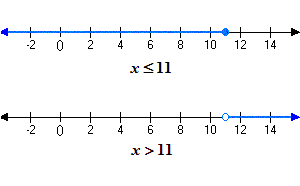# Inequalities

Inequalities are mathematical sentences comparing two quantities that are not equal (or possibly not equal). There are five inequality symbols:

 $x\ne 3$ $x$ is not equal to $3$ $x<3$ $x$ is less than $3$ $x>3$ $x$ is greater than $3$ $x\le 3$ $x$ is less than or equal to $3$ $x\ge 3$ $x$ is greater than or equal to $3$

## Graphing Inequalities in One Variable

The solutions of inequalities can be graphed on the number line as rays . If the inequality is "strict" ( $<$ or $>$ ), we use an open dot to indicate that the endpoint of the ray is not part of the solution. For the other types of inequalities ( $\le$ and $\ge$ ), we use a closed dot to indicate that the endpoint is part of the solution.

Example:See also compound inequalities and solving linear inequalities .